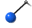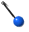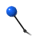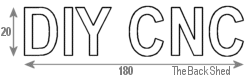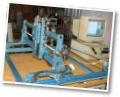Building my CNC Router

 >> Home Page >> CNC explained >> Building my own CNC table >> Info >> Photo Gallery >> Useful Links

Ohms Law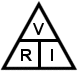V = Volts
R = Resistance (ohms,)
I = Current ( Amps)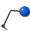Formular Example V = R * I 5* 2A = 10Vs I = V / R 10V / 5= 2A R = V / I 10V / 2A = 5Watts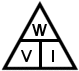W = Watts
V = Volts
I = Current ( Amps)

 Formular Example W = V * I 10V * 2A = 20W I = W / V 20W / 10V = 2A V = W / I 20W / 2A = 10V W = R * I2 5* 22 = 20W W = V2 / R 10V2 / 5= 20W R = W / I2 20W / 2A2 = 5R = V2 / W 10V2 / 20W = 5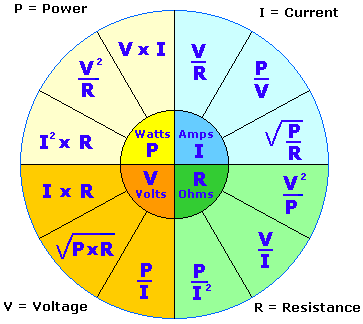AC Theory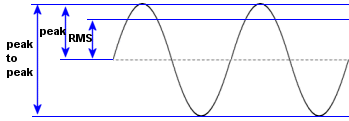RMS 0.707 Peak voltage

Impedance

AC current in a capacitive or inductive component. Impedance is the resistance ( measured in ohms ) of a reactive component ( capacitor, inductor ) at a certain AC frequency.

 Capacitor Inductor Xc = 1 / ( 2 * Pi * f * C ) Xl = 2 * Pi * f * L where Pi = 3.14 where Pi = 3/14 f = frequency in Hz f = frequency in Hz C = capacitance in Farads L = Inductance in Henry eg A 100uF cap at 50Hz eg A 20mH inductor at 50Hz Xc = 1/(2*3.15*50Hx*0.0001F) Xl=2*3.14*50Hx*0.02 Xc = 31.8 ohms Xl = 6.28 ohms

Multipliers.

 Pica 0.000,000,000,001 1^-12 Nano 0.000,000,001 1^-9 Micro 0.000,001 1^-6 Milli 0.001 1^-3 Centi 0.01 1^-2 Unit 1 1 Kilo 1,000 1^3 Mega 1,000,000 1^6 Giga 1,000,000,000 1^9 Tera 1,000,000,000,000 1^12

The Metric system.

All measurements are based on a unit, and a multiplier, like those above. ie for distance we use a measure called the meter, and thats the only unit of distance we need to remember. We also use a multiplier, so we call 1000 meters a KiloMeter, or 1/1000 of a meter is a MilliMeter. Easy.

Another easy one to remember is Centigrade as a measurement of temperature. Water freezes at 0 degrees, and boils at 100 degrees. Body temperature is about 32 degrees C.

Some common conversions

 To Convert To Mulitply by Inches MilliMeters 0.03937 MilliMeters Inches 25.4 Feet Meters 3.281 Meters Feet 0.3048 Miles KiloMeters 1.609 KiloMeters Miles 0.6214

For more conversions, visit http://www.simetric.co.uk/si_c.htm

Common copper wire sizes and resistance

 AWG Max I * Inch Dia mm Diaper 1000' **per km ** 22 0.918 0.025 0.644 16.46 54 20 1.46 0.032 0.812 10.35 33.95 18 2.32 0.04 1.024 6.51 21.36 16 3.69 0.05 1.291 4.09 13.42 14 5.87 0.064 1.628 2.57 8.43 12 9.33 0.08 2.053 1.62 5.31 10 14.8 0.101 2.588 1.02 3.34 8 23.6 0.128 3.264 0.64 2.1 6 37.5 0.162 4.115 0.4 1.31 4 59.6 0.204 5.189 0.25 0.83 2 94.8 0.257 6.544 0.16 0.52 1 119 0.289 7.348 0.12 0.39 * max safe current in a tightly wound coil ** at 25 degrees C Source: The Radio Amatures Handbook 1978

Resistance of other materials compared to copper
where copper = 1

 Aluminium : 1.6 Brass : 3.7 - 4.9 Gold : 1.4 Silver : 0.94 Iron : 5.68 Lead : 12.8 Steel : 7.6 - 12.7 Tin : 6.7 Zinc : 3.4 Nickel : 5.1 Source: The Radio Amatures Handbook 1978